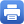Columbia Home
 Home » Programs » Undergraduate Program » Linear Algebra » Linear Algebra & ProbabilityLinear Algebra & ProbabilityMATH UN2015 – Linear Algebra & Probability MATH UN2015 features linear algebra with a focus on probability and statistics. The course covers the standard linear algebra topics: systems of linear equations, matrices, determinants, vector spaces, bases, dimension, eigenvalues and eigenvectors. It also teaches applications of linear algebra to probability, statistics and dynamical systems giving a background sufficient for higher level courses in probability and statistics. The topics covered in the probability theory part include conditional probability, discrete and continuous random variables, probability distributions  and the limit theorems, as well as Markov chains, curve fitting, regression, and pattern analysis. Both linear algebra and probability theory provide concepts that are central to most areas of mathematics and statistics. These concepts are also used in many natural sciences as well as in the fields of engineering and data science. MATH S2015 is a course best suited for students who wish to learn how concepts in abstract mathematics are used in such applications. In addition to introducing the main concepts and results from linear algebra and probability theory, the course has a project component that focuses on applications to natural and social sciences. No a priori background in the life sciences or social sciences is assumed and no previous programming experience is required. MATH UN2015 vs MATH UN2010 MATH UN2015 – Linear Algebra & Probability is a course best suited for students who wish to focus on applications and practical approach to problem solving, rather than abstract mathematics and mathematical proofs. It is recommended to students majoring in engineering, technology, life sciences, social sciences, and economics. Math majors, joint math majors, and math concentrators should take MATH UN2010 – Linear Algebra, which focuses on linear algebra concepts and theoretical foundations that are needed for upper-level math courses. Students will not receive credit for both courses UN2010 and UN2015.  Prerequisites MATH UN1101 – Calculus I (or equivalent) is strongly recommended. Textbooks: Required: Deisenroth, M. P., Faisal, A. A., & Ong, C. S. (2020). Mathematics for Machine Learning. Cambridge University Press.* Barron, E. N., & Del Greco, J. G. (2020). Probability and statistics for STEM: a course in one semester. Springer International Publishing.* Optional (recommended): Bretscher, O. (2019). Linear algebra with applications. (5th ed.). Pearson Education. (Publisher link) Unpingco, J. (2020). Python For Probability, Statistics, And Machine Learning. (2nd ed.). Springer Nature.* (Code available on GitHub). Note: The materials marked with * are available in electronic format at no cost for Columbia/Barnard students. Topics The sequence of topics covered is as follows. Linear Algebra Systems of linear equations. Matrices and vectors. Gaussian elimination. Vectors spaces and subspaces. Linear independence. Basis and dimension. Linear transformations. Matrix representation. Image and kernel of a linear transformation. Inner products. Length and distances. Angles and orthogonality. Orthonormal basis, orthogonal complement, orthogonal projections. Determinant and trace. Eigenvalues and eigenvectors. Spectral theorem. Singular value decomposition. Rank k approximations. Probability Basic notions from probability theory. Conditional probability, Bayes’ theorem. Random variables. Probability distributions. Summary statistics. Moment generating functions. Joint distributions. Independent rvs. Covariance and correlation. Central limit theorems. Conjugacy and exponential family. Change of variables/inverse transform. Some applications Markov chains, network flow, economic models, differential equations. Least squares and data fitting, linear regression. Principal component analysis. Dimensionality reduction.Print this page